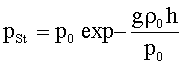# What is H in Bernoulli's equation

## The energy balance then changes into Bernoulli equation

The Bernoulli equation

If there is no friction, the principle of maintaining the mechanical Energy: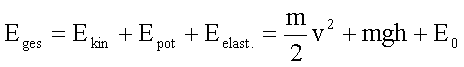By relating the energy to the unit of volume, we move on to energy density or pressures: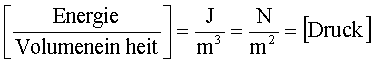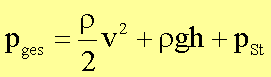The total pressure is the sum of Back pressure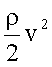,Gravity pressure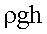andstatic pressure pSt..

If the density is a function of the pressure, then more generally applies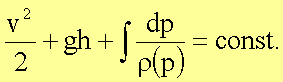For an ideal gas, the relationship between pressure and density in the isothermal case is described by Boyle’s law:

Boyle’s Law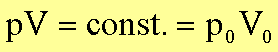With the molar volume VM. and the molar mass µ is obtained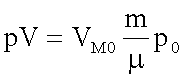or.Under normal conditions (not standard conditions) the following applies:

 T0 V.M0 p0 r0(Air) 0 ° C = 273.15 K 22.4 l 1,01325.105 Pa 1.29 kg / m3

The following applies to an ideal gas: At a given temperature, the density of a gas is proportional to its pressure.

In the following we are interested in the pressure in a non-flowing liquid or gas column, i.e. with v = 0 the following applies: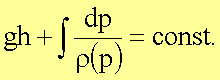For an incompressible liquid with ptotal (h = 0) = p0 then applies to the static pressure: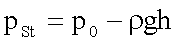The static pressure of a column of liquid decreases with increasing height.

In the case of a gas, the following applies (as an approximation of an ideal gas) at constant temperature (isothermal stratification):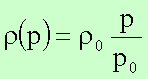The calculation of the integral in the Bernoulli equation gives: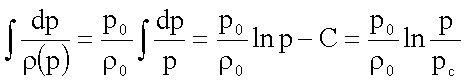At the height h = 0, let the pressure ptotal = pSt. = p0 and the density r0. From this it follows for the constant of integration pc = p0/ e. The barometric altitude formula results for the altitude dependence of the static pressure: